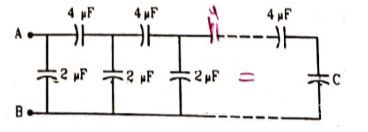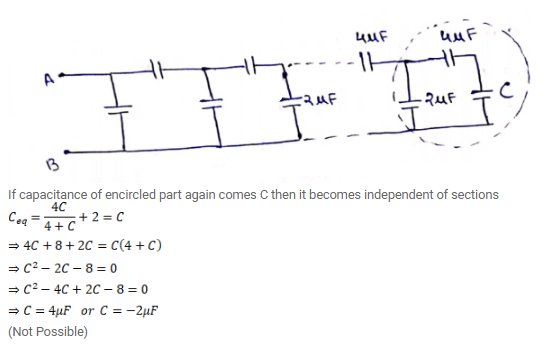# A finite ladder is constructed by connecting several sections ofQuestion:

A finite ladder is constructed by connecting several sections of $2 \mu \mathrm{F}, 4 \mu \mathrm{F}$ capacitor combination as shown in figure. It is terminated by a capacitor of capacitance $C$. What value should be chosen for $C$, such that the equivalent capacitance of the ladder between the points $A$ and $B$ becomes independent of the number of sections in between?Solution: